### Contingency Table, Scatterplot & Pearson’s r

#### Contingency Table:

It is very similar to a frequency table. But the major difference is that a frequency table always concerns only one variable, whereas a contingency table concerns two variables.

If you want to know the relationship between two ordinal or nominal variables then you have to look for contingency table which displays you this relationship.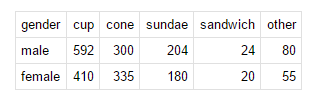For example, the above contingency table has two rows and five columns and shows the results of a random sample of   adults classified by two variables, namely gender and favorite way to eat ice cream. One benefit of having data presented in a contingency table is that it allows one to more easily perform basic probability calculations, a feat made easier still by augmenting a summary row and column to the table.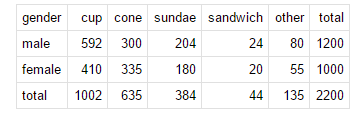The above table is an extended version of the first table obtained by adding a summary row and column. These summaries allow easier computation of several different probability-related quantities. For example, there’s a  1002 / 2200 = 45.54 % probability that the person sampled prefers their ice cream in a cup, while the probability that a random participant is female is 1000/2200 = 45.54 % . What’s more, computing conditional probabilities is made easier using contingency tables, e.g., the probability that a person prefers ice cream sandwiches given that the person is male is 24/2200 = 2% , while the conditional probability that a person is male given that ice cream sandwiches are preferred is  24/44= 54.54 %. These things are called conditional proportion and marginal proportion.

You can calculate column percentage for each variable like the example above.

Column percentage= cell / total (column) *100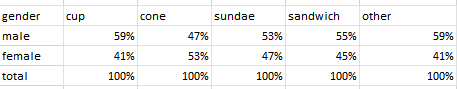Now from this table you can see if two variables are correlated or not.

#### Quantitative Variable and Scatter plot:

A contingency table is useful for nominal and ordinal variables, but not for quantitative variables. For quantitative variables, a scatterplot is more appropriate.

Scatter plot displays relation between two quantitative variables exploratory variable will be in X axis and Response variable will be in y axis.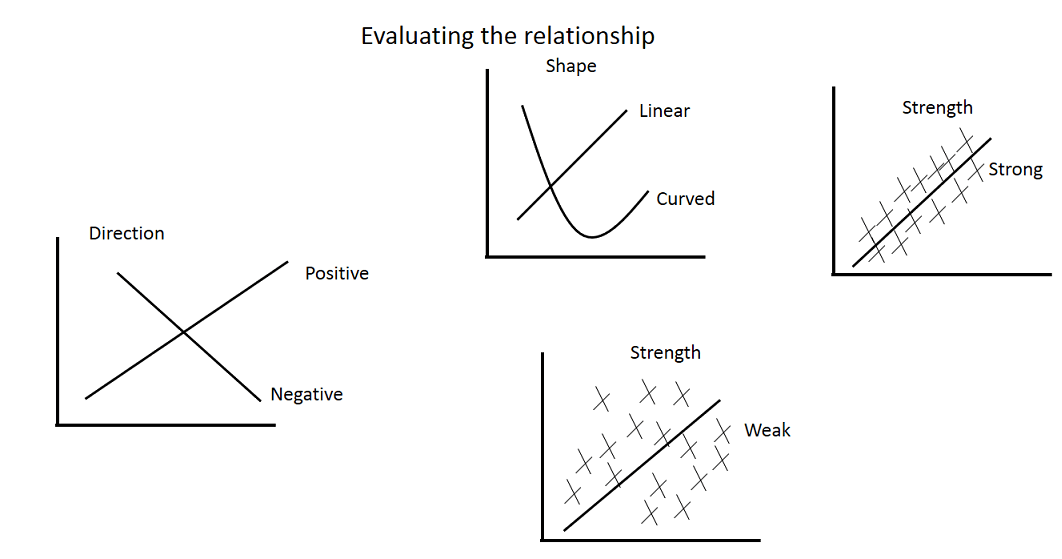So, we can display relationships between two variables by means of tables and graphs. When the variables in a study are measured on a nominal or ordinal level we use a contingency table and when they are measured on a quantitative level we use a scatterplot.

#### Pearson correlation or Pearson’s r:

• scatterplot shows at a glance the relationship between two quantitative variable if you plot independent variable on the horizontal x-axis and dependent variable on the vertical y-axis. But now the question is how strong is this correlation? Pearson’s r express the strength of the correlation.
• One of the most important advantages of Pearson’s r is that it expresses the direction and strength of the linear correlation between two variables with a single number.
• A positive Pearson’s r indicates that a correlation is positive, and a negative correlation indicates that it is negative. The size of r expresses how tightly the observations are clustered around the imaginary best-fitting straight line through the cloud of data.
• Pearson’s r is always a number between -1 and 1. -1 refers to a perfect negative correlation. + 1 to a perfect positive correlation. And 0 means that there is no correlation is there.#### How to compute the Pearson’s r?

First change all original scores to z-scores. In other words, standardize the values. The reason is that we want the Pearson’s r to be a number between minus 1 and 1. If we don’t standardize, the measure of correlation will be expressed according to the original metrics. To standardize calculate mean and Standard deviation.

Here is the formula to compute Pearson’s R.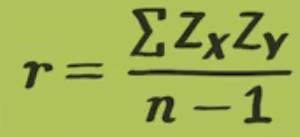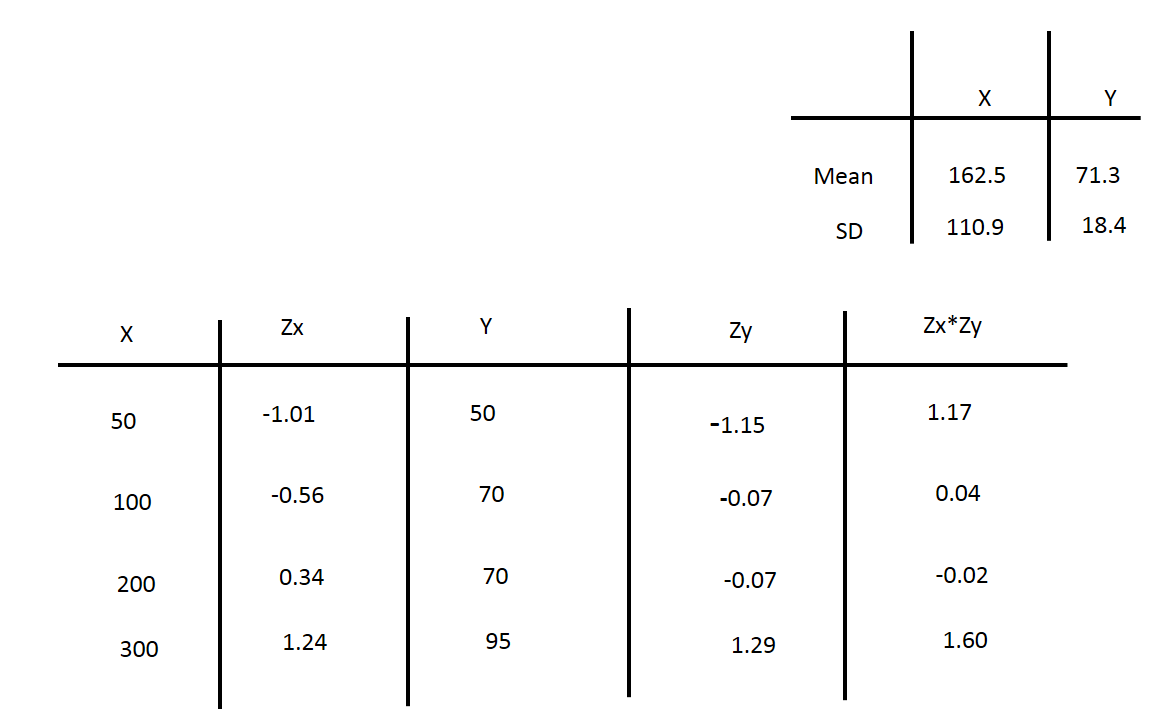Now if you apply the above fomula to the last column then you will get Sum =2.78

r= 2.78 / 4-1

= 0.93

##### What does it mean by r = 0.93?

It means there is strongly linear relationship between x and y.

Before going to calculate Pearson’s r first see the Scatterplot. If there is no linear relation then no Pearson’s r. See the below diagram it’s curvilinear relation so there is no strong Pearson’s r.So, as a summary, A scatterplot helps us to broadly assess whether a correlation is strong or weak, but it does not tell us exactly how strong the relationship is. Pearson’s r is a measure that can show us exactly that.